Posts

Power Supply For Students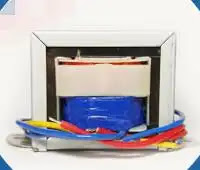Power Supply For Students | Design Your Own Power Supply

My First Project As A Student Of Electronics Engineering - Design Power SupplyWhen I was a student of Electronics engineering, this was the first task, that is to design a power supply unit. It might be your first project during first semester of ECE discipline as well. We are going to design a variable power supply with maximum output voltage of 24V.
Project Goals & Outline: What is power supply unit and why it is important? Block diagram and explain each block Circuit diagram and explanation of each component Choosing the right components Specifically designed calculators, which helps in selecting right capacitance and resistance
After thoroughly reading this lesson you will able to design a regulated and variable power supply of desired value. I have designed my own calculator, you can calculate the capacitors value (filtering or smoothing capacitor), resistors values (for LM317) according to your need. You can able to selec…

Voltage Divider Rule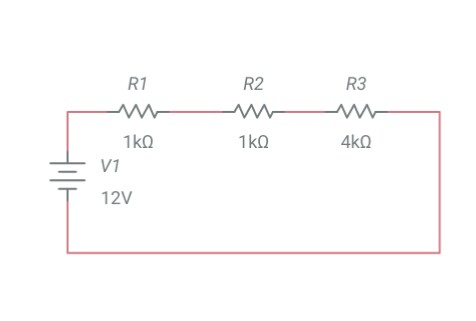In this tutorial I am going to introduce a top-notch technique for solving series circuits. You can solve a series circuit easily by applying Ohm's law. But Ohm's law has a limitation. You have to know the current flowing through the circuit. This method allows you to find out the voltage across any series component without knowing the current. This method is derived from two very common circuit solving laws, that is Ohm's law and KVL. In this article I am going to derive the expression of voltage divider rule as well.
Explanation:It is only applicable to series circuits where current remains same throughout the circuit. Consider a series circuit given below.

Let,
v1 = voltage across R1 v2 = voltage across R2 v3 = voltage across R3 i = total current across the circuit
According to KVL,
v = v1 + v2 + v3 v = iR1 + iR2 + iR3 v = i (R1 + R2 + R3) i = v/ (R1 + R2 + R3) ….. equation 1
If you want to evaluate voltage across R2, then using Ohm's law
v2 = iR2 i = v2/R2 ….. equation 2
Look …

Series Resistors | Equivalent Series Resistance | Formula Of Series Resistance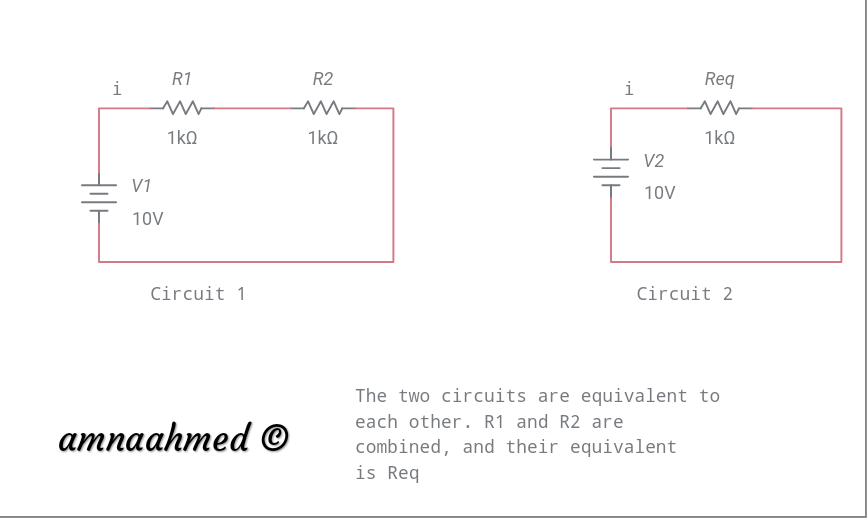Resistors In Series & Evaluation Of Equivalent Series Resistance

Current & Voltage Through Series Resistors:

Before starting my tutorialon seriesconnectedresistors, I would like to recall series circuits. “Two elements connected in such a way that they share a single node exclusively.”
Similarly, two resistors are connected in series, when they share a single node exclusively. Properties Of Series Connected Resistors:Current remains same in every series connected resistor Another property of series circuit is the voltagedivision property. The voltage divided proportionally to all resistors. The larger the resistance value, the greater will be the voltage drop across that resistance. Formula Of Resistance In Series:Apply Ohm's law to the circuits below,
Circuit 1:
Voltage drop across R1 is va va = iR1
Voltage drop across R2 is vb vb = iR2
Total voltage across circuit the is the sum of voltage drops across R1 and R2 v1 = va + vb … equation 1 Circuit 2:
Voltage across Req v2 = iReq … equa…
Work Energy & Power
Power Dissipation In Resistors | Applications Of Ohm's Law | Work Energy & Power:Ohm's law is applicable in determining the power dissipation in resistive elements.
Energy:Energy and work has same units, that is joules. Energy is defined as ability to do work. There are many forms of energy, like electrical, mechanical, kinetic thermal and potential. Electricity is a form of energy. Energy can change from one form to another willresultin heat, which is also important form of energy, when you study electronics and electrical engineering. The change of energy from one form to another is called energy transformation. Heat changes electrical properties of materials. A battery is a source of electrical energy and has the ability to do work.
Energy = Power*Time Work:Work is defined as expenditure of energy. The amount of work done is the amount of energy transformed. Power:Power is rate of change of energy or rate of energy transformation or the rate at wh…

Best Electronic Components Kits - Electronic Component Storage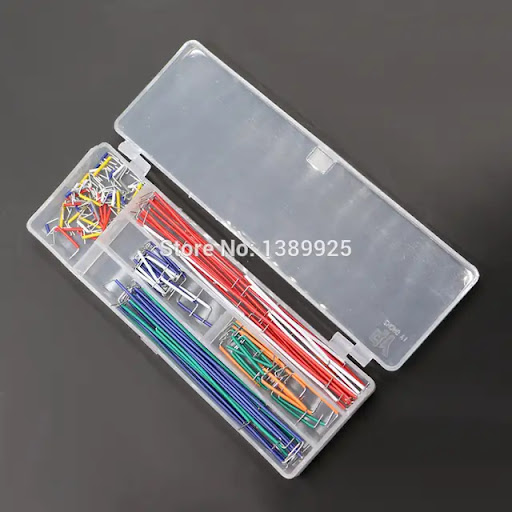Whether you are a student, hobbyist, amateur or a professional, it is a good practise to keep your things properly, neat and clean. In this article I will explain the way in which you maintain your discrete electronic components.
As a female I used to keep my things properly, in a well organiser manner. I have a limited space, where I put all my stuff. So I am always looking for organiser, shelves. The purpose of organising your stuff is to store your components, prevent them from dust, keep it away from kids, and you get them back easily when you need them.

After a lot of search, I found this kit. I like it very much. Now look at it!!

Starter Kit for Resistor /LED / Capacitor / Jumper Wires / Breadboard resistor Kit with Box
It seems decent, and economical as well. It is really good for storing discrete electronic components like diodes, resistors, capacitors, transistors, different ICs etc.
“Color is…

Ohm's Law - Statement, Explanation, Calculations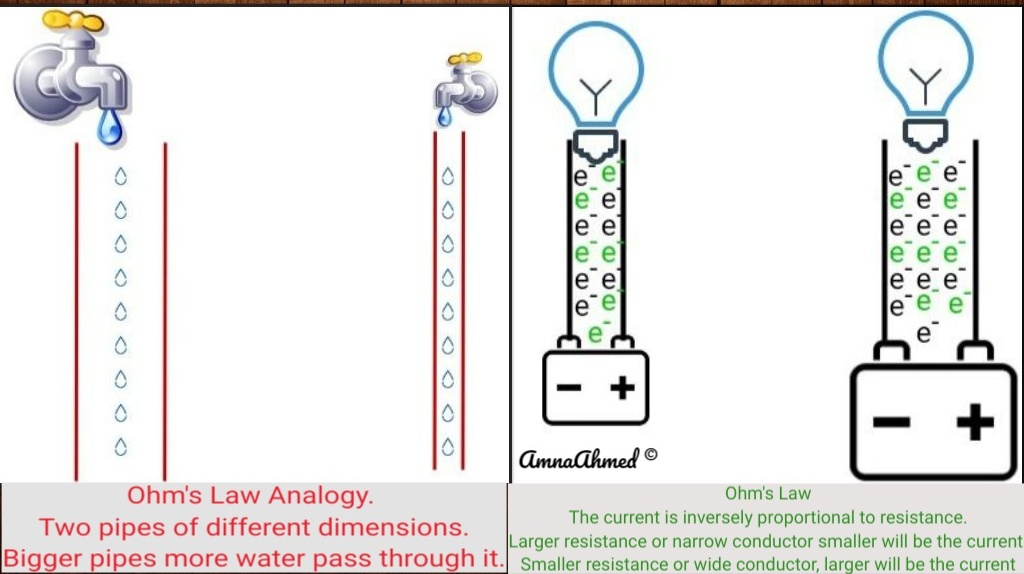This is the very first law related to electricity. It was presented by German physicist Georg Simon Ohm (1787 - 1854). The importance of this law is evident from the fact that it is still valid and used in almost all the design, troubleshooting and repairing of any electrical system. It is applicable to all linear circuits (a circuit is linear if voltage and current graph is a straight line).  It is also applicable to both AC or DC circuits. It describes the relationship between three basic quantities, that is, current, voltage and resistance.
The scientist performed series of experiments, at that time there were no electrical measuring instruments. In today's world we can easily verify Ohm's law because we have measuring instruments.
The empirical law describes the linear relationship between current and voltage. “At constant temperature, it takes one volt of electrical pressure (voltage) to push one ampere of current through one ohm resistor”
OR “At constant temperature, current…

Construction of Resistors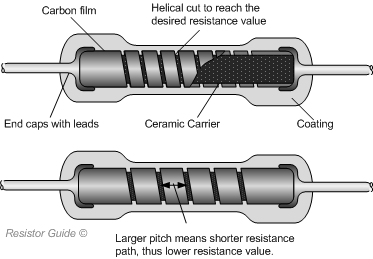What is inside a resistor
Construction of Resistors - Some basic typesOutline: Wire wound resistorsCarbon composition resistorsCarbon film resistorsMetal film resistors

What is inside the wire wound resistors? Wire wound resistors are the oldest types of resistors. They are suitable for high power and high precision applications. You can imagine the structure of resistor by its name, a spiral helix wound on a tube.
A resistant wire (made from alloy) with high resistivity value is wounded on an insulated core such as ceramic or glass. The value wire wound resistor is determined by this formula, R = ρ*L/A
The larger the wire the larger the resistance. the larger the cross sectional area, the lower the resistance. To make larger resistance a large thin wire is required.
Pure metals have high temperature coefficient because they are more like to change their resistances as temperature changes. (Effect of temperature on resistance of a conductor). Metal alloys are used because they low temperat…